American Journal of Theoretical and Applied Statistics
Volume 5, Issue 3, May 2016, Pages: 154-161

Parameter Estimation of Kumaraswamy Distribution Based on Progressive Type II Censoring Scheme Using Expectation-Maximization Algorithm

Wafula Mike Erick*, Kemei Anderson Kimutai, Edward Gachangi Njenga

Department of Statistics and Actuarial Science, Kenyatta University (KU), Nairobi, Kenya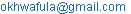(Wafula M. E.)

*Corresponding author

Wafula Mike Erick, Kemei Anderson Kimutai, Edward Gachangi Njenga. Parameter Estimation of Kumaraswamy Distribution Based on Progressive Type II Censoring Scheme Using Expectation-Maximization Algorithm. American Journal of Theoretical and Applied Statistics. Vol. 5, No. 3, 2016, pp. 154-161. doi: 10.11648/j.ajtas.20160503.21

Received: May 3, 2016; Accepted: May 23, 2016; Published: June 1, 2016

Abstract: This project considers the parameter estimation problem of test units from Kumaraswamy distribution based on progressive Type-II censoring scheme. The progressive Type-II censoring scheme allows removal of units at intermediate stages of the test other than the terminal point. The Maximum Likelihood Estimates (MLEs) of the parameters are derived using Expectation-Maximization (EM) algorithm. Also the expected Fisher information matrix based on the missing value principle is computed. By using the obtained expected Fisher information matrix of the MLEs, asymptotic 95% confidence intervals for the parameters are constructed. Through simulations, the behaviour of these estimates are studied and compared under different censoring schemes and parameter values. It’s concluded that for an increasing sample; the estimated parameter values become closer to the true values, the variances and widths of the confidence intervals reduce. Also, more efficient estimates are obtained with censoring schemes concerned with removals of units from their right.

Keywords: Kumaraswamy Distribution, Progressive Type II Censoring, Maximum Likelihood Estimation, EM Algorithm

Contents

1. Introduction

Censored sampling arises in a life testing experiment whenever the experimenter does not observe (either intentionally or un-intentionally) the failure times of all units placed on a life test.

"According to Horst, a data sample is said to be censored when, either by accident or design the value of the variables under investigation is unobserved for some of the items in the sample." Inference based on censored sampling has been studied during the past over 50 years by numerous authors for a wide range of lifetime distributions.

In this study, we assume that the lifetimes have Kumaraswamy distribution. This distribution was introduced by Kumaraswamy as a probability density function for double bounded random processes.  This distribution is applicable to many natural phenomena whose outcomes have lower and upper bounds, such as the heights of individuals, scores obtained on a test, atmospheric temperatures, hydrological data etc.

The two parameter Kumaraswamy distribution has a PDF and CDF given respectively by;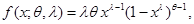(1)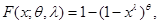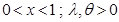(2)

Kumaraswamy and Ponnambalam et al. [2, 3] have pointed out that depending on the choice of the parameters, this distribution can be used to approximate many distributions, such as uniform, triangular, or almost any single model distribution and can also reproduce results of beta distribution. The basic properties of the distribution have been given by Jones. 

Inferential issues for the Kumaraswamy distribution based on censored data have been addressed by Gholizadeh et al.  who considered the Bayesian estimation of Kumaraswamy distribution under progressively Type II censored samples. Tabassum et al.  explored the Bayesian analysis of Kumaraswamy distribution under failure censoring sampling scheme. Feroze et al  estimated the parameters of Kumaraswamy distribution under progressive type II censoring with random removals using maximum likelihood method.

Most recently, Mostafa et al  derived parameter estimators of Kumaraswamy distribution based on general progressive type II censoring scheme using maximum likelihood and Bayesian approaches. Also, some of the recent work on progressive censoring include but not limited to [9-14]. As far as we know, no one has described the EM algorithm for determining the MLEs of the parameters of the Kumaraswamy distribution based on progressive type-II censoring scheme.

The purpose of this study is to estimate the shape and scale parameters of the Kumaraswamy distribution under progressive type-II censoring using the EM algorithm and to compare the results under different censoring schemes.

In this work, we propose to use EM algorithm for computing MLEs. This is because the EM algorithm is relatively robust against the initial values compared to the traditional Newton-Raphson (NR) method. [15, 16] For some of the recently relevant references on EM algorithm and censoring include [17 and 20].

2. Parameter Estimation

2.1. Progressive Type II Censoring

Suppose n identical units are put on a test and the lifetime distributions of the n units are denoted by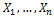.

The integer m < n is ﬁxed at the beginning of the experiment and they are the units which are observed completely until failure.

The censoring occurs progressively in m stages. These m stages offer failure times of the m completely observed units. At the time of the first failure (the first stage),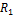of the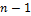surviving units are randomly withdrawn from the experiment. At the time of the second failure (the 2nd stage),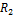of the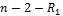surviving units are withdrawn and so on. Finally, at the time of the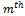failure (thestage), all the remaining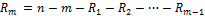surviving units are withdrawn. According to Childs and Balakrishnan, we refer to this as progressive Type-II right censoring with scheme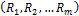. 

2.2. Maximum Likelihood Estimation

Let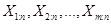denote a progressive Type II censored sample from Kumaraswamy distribution. Then according to  the likelihood function based onprogressively Type II censored sample is given by;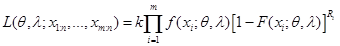(3)

From equations (1) and (2), the likelihood function based on progressive Type II censored sample is as follows;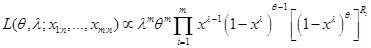(4)

The log-likelihood function of equation (4) can be written as follows: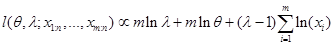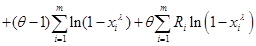(5)

2.3. EM Algorithm

We propose the EM algorithm, introduced by Dempster et al.  to find the MLEs.

Let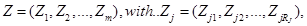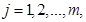be the censored data.

We consider the censored data as missing data. The combination (X, Z) = W forms the complete data set. The log-likelihood function based on W can be written respectively as: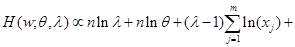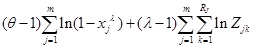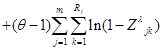(6)

In the E-step, one requires to compute the pseudo-likelihood function. This can be obtained from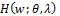by replacing any function of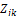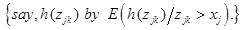Therefore equation (6) becomes;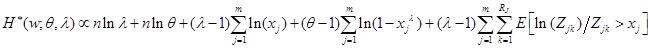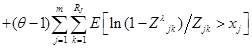(7)

Therefore,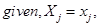the conditional distribution of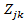follows a truncated Kumaraswamy distribution with left truncation at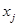. That is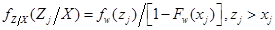(8)

Therefore the conditional expectations in equations (6) and (7) can be obtained as follows: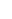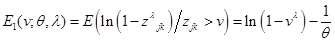(9)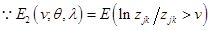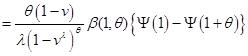(10)

Thus, in the M-step of the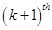iteration of the EM algorithm, the value of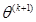is first obtained by solving the following equation: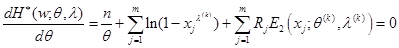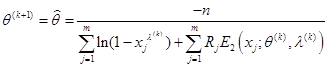(11)

Onceis obtained,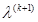is obtained by solving the equation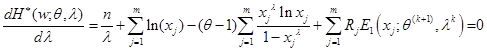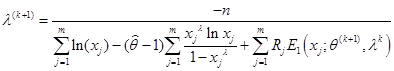(12)

2.4. Asymptotic Variance-Covariance Matrix of the MLEs

The variance–covariance matrix is used to provide a measure of precision for parameter estimators by utilizing the log-likelihood function. We first compute the variance–covariance matrix of parameters θ and λ by considering a complete data set from the Kumaraswamy distribution.

For such a case, the log likelihood function based on X is obtained as follows;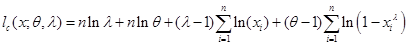(13)

Using equation (13), the Fisher information matrix for the complete data set is given as;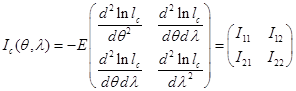And the variance-covariance matrix of parameters θ and λ is given by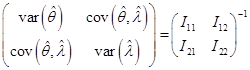(14)

Where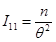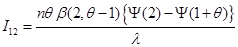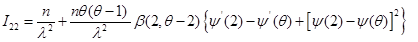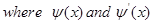are the digamma and trigamma functions respectively.

In this work, we are interested in deriving the asymptotic variance–covariance matrix for the MLEs based on the EM algorithm. For this we will use the procedure that was established by Louis and Tanner. [23, 24] The idea of this procedure is given by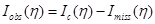(15)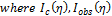and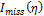denote the complete, observed, and missing (expected) information, respectively, and η = , λ). The Fisher information matrix for a single observation which is censored at the time of the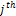failure is given by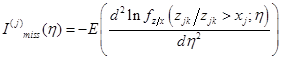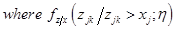is given in Equation (8). The expected values of the second partial of the log-likelihood function of Z given X are calculated as follows;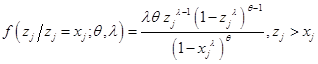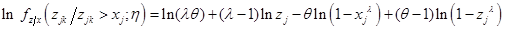(16)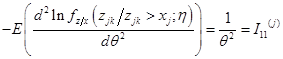(17)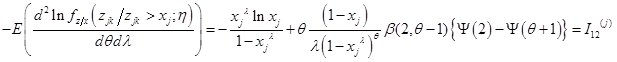(18)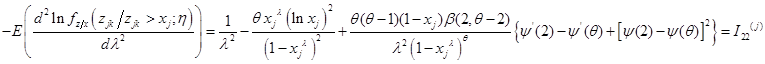(19)

Note that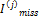is a function of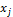and η, since the expectation is taken with respect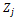; therefore, the expected information matrix is simply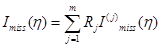(20)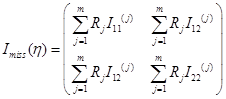Hence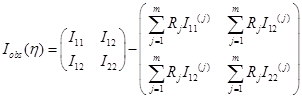Therefore, the variance–covariance matrix of parameter η can be obtained by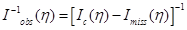(21)

Using equation (21) an approximate 100(1−α) % confi-dence intervals for θ and λ is obtained respectively, as;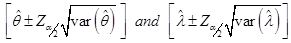(22)

Where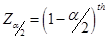is the percentile of the standard normal distribution.

3. Numerical Results and Discussion

In this section a simulation study is conducted to investigate how the above estimators perform in estimating the parame-ters of Kumaraswamy distribution based on progressive type II censored data. The samples were generated based on the algorithms of Balakrishnan and Sandhu and Aggarwala and Balakrishnan (1998). [25, 26] The censoring schemes con-sidered are given in table 1 below;

Table 1. Censoring schemes.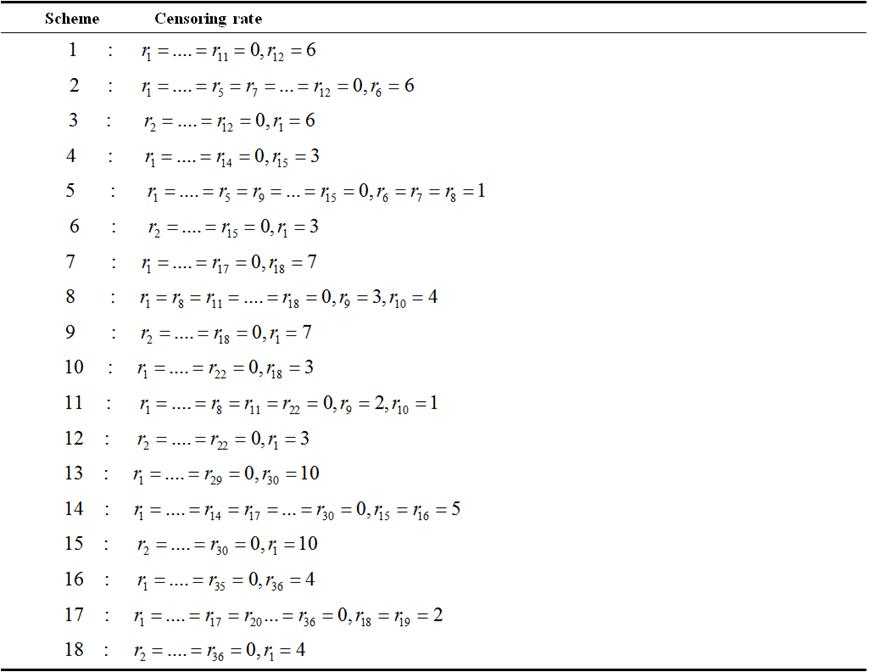Clearly from table 1, schemes 1, 4, 7, 10, 13 and 16 are right censored schemes; 2, 5, 8, 11, 14 and 17 are centre censored while 3, 6, 9, 12, 15 and 18, are left censored schemes. The right, centre and left censored schemes are respectively denoted as n:m-R, n:m-C and n:m-L.

All the computational results were computed using R software

Table 2. MLEs, variances and confidence intervals of MLEs of Kumaraswamy distribution when λ=0.6 and θ=1.0.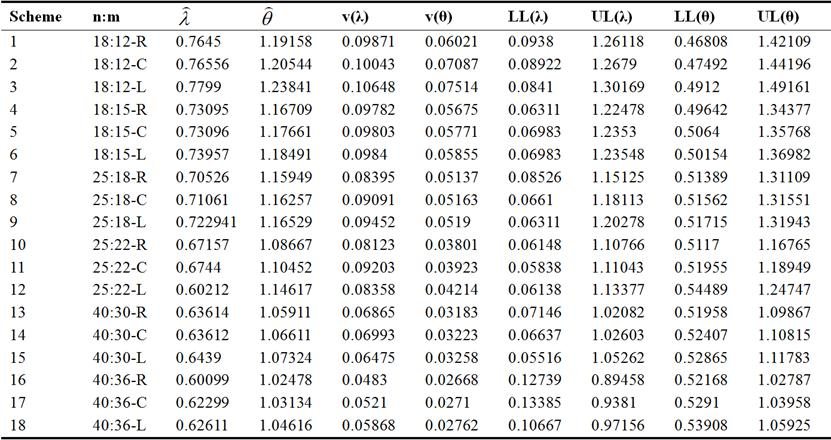From table 2, it is observed that irrespective of the censoring rate and the position at which the censored units are removed from the sample, for increasing sample size;

(i) the estimated value of the parameter becomes closer to the true value,

(ii) the variances of the MLEs decrease

Table 3. Effect on the Confidence intervals of the estimates.

 Scheme n:m width of λ width of θ 1 18:12-R 1.16738 0.95301 2 18:12-C 1.17868 0.96704 3 18:12-L 1.21759 1.00041 4 18:15-R 1.16167 0.84735 5 18:15-C 1.16305 0.85614 6 18:15-L 1.16547 0.86382 7 25:18-R 1.06599 0.7972 8 25:18-C 1.11503 0.79989 9 25:18-L 1.13967 0.80228 10 25:22-R 1.04618 0.65595 11 25:22-C 1.05205 0.66994 12 25:22-L 1.06329 0.70258 13 40:30-R 0.94936 0.57909 14 40:30-C 0.95966 0.58408 15 40:30-L 0.99746 0.58918 16 40:36-R 0.76719 0.50619 17 40:36-C 0.80428 0.51048 18 40:36-L 0.86489 0.52017

Table 3 clearly shows that the widths of 95% confidence intervals tend to be lesser as the sample size increases.

Table 4. Effect of the number of censored units on estimates.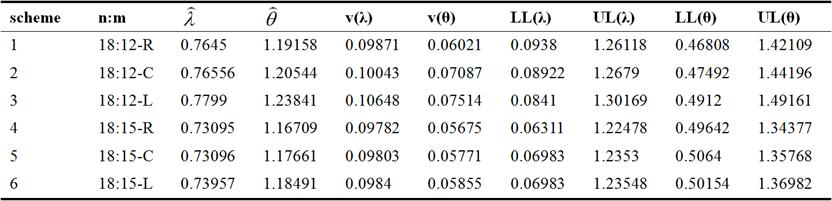Table 4 has been extracted from table 2, so as to clearly illustrate the effect of censored units on the parameter estimates. The results in table 4 show that when the sample size is kept constant, then better estimates are obtained when the censored units are reduced. Schemes 4-6 have better estimates compared to schemes 1-3 because the number of censored units in schemes 4-6 are each 3 units while in schemes 1-3, we have 6 units censored from each.

Table 5. Effect of position of removal of units in the scheme on estimates.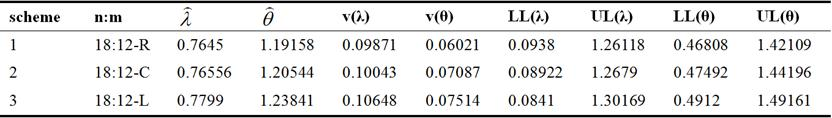The removal of units in scheme 1, 2 and 3 was done at the 12th, 6th, and 1st failures respectively and from the results it was observed that scheme 1 which is right censored, gave a better estimate followed by scheme 2 (centre censored scheme) and lastly scheme 3 (left censored scheme). The same trend was observed across all the censoring schemes i.e all the right censored schemes resulted in better estimates followed by centre censored and left censored in that order.

Table 6. MLEs, variances and confidence intervals of MLEs of Kumaraswamy distribution when λ=2.2 and θ=3.5.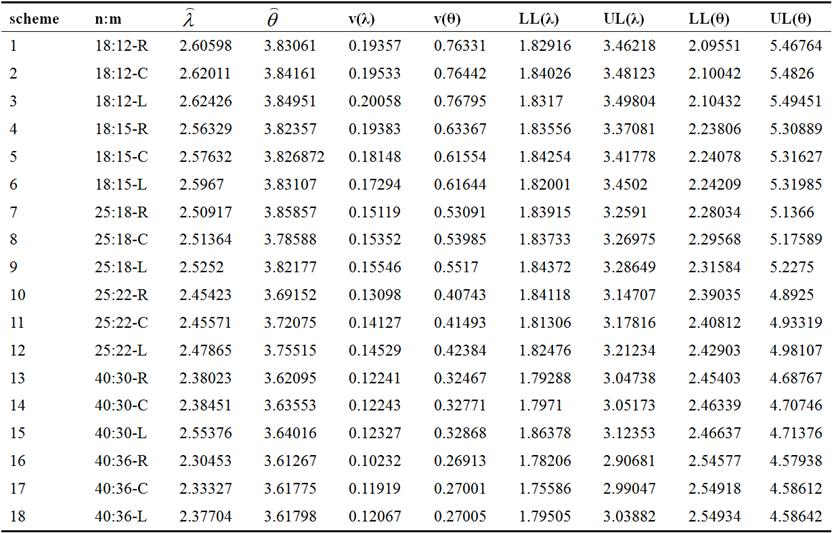Table 6 also shows that for increasing sample size the estimated value of the parameter becomes closer to the true value and the variances of the MLEs decrease.

However, these variances are much higher than those obtained in table 2.

Table 7. Effect on the Confidence intervals of the estimates.

 Scheme n:m width of λ width of θ 1 18:12-R 1.163302 3.37213 2 18:12-C 1.64097 3.38218 3 18:12-L 1.66704 3.39019 4 18:15-R 1.53525 3.07083 5 18:15-C 1.57524 3.07549 6 18:15-L 1.6302 3.07776 7 25:18-R 1.41985 2.85626 8 25:18-C 1.43242 2.88021 9 25:18-L 1.44277 2.91166 10 25:22-R 1.30589 2.50215 11 25:22-C 1.3651 2.55204 12 25:22-L 1.38758 2.52507 13 40:30-R 1.2545 2.23364 14 40:30-C 1.25463 2.24407 15 40:30-L 1.25975 2.24739 16 40:36-R 1.12475 2.03361 17 40:36-C 1.23461 2.03694 18 40:36-L 1.24377 2.03708

The widths of the confidence intervals are also higher under these set of parameter values and tend to be lesser for an increasing sample size.

Table 8. Effect of the number of censored units on estimates.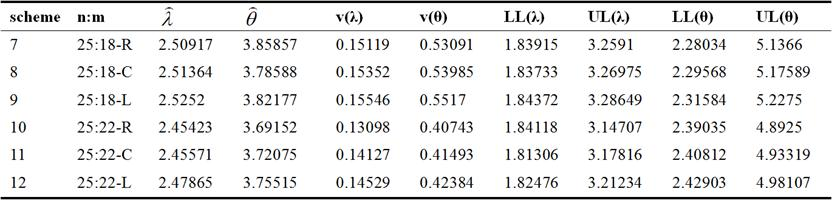Table 6 as well reveals that reducing the censored units leads to better estimates for a constant sample size. In schemes 7-9, the number of units censored are each 7, while in schemes 10-12, the censored units are each 3 and we see from the results that schemes 10-12 gave better estimates compared to schemes 7-9.

Table 9. Effect of position of removal of units in the scheme on estimates.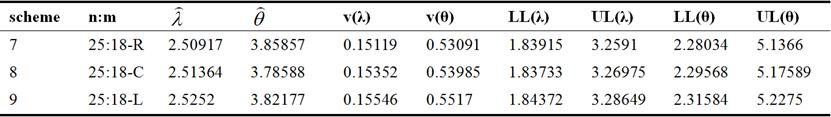The removal of units in scheme 7, 8 and 9 was done at the 18th, 9th and 10th, and 1st failures respectively and from the results it was observed that scheme 7, gave a better estimate followed by scheme 8 and finally scheme 9. This trend was observed to cut across all the censoring schemes i.e all the right censored schemes resulted in better estimates followed by centre censored and left censored in that order.

4. Conclusion

This study has addressed the problem of estimation of parameters of the Kumaraswamy distribution based on progressive Type-II censored data. It is shown that the MLEs of the scale and shape parameters can be obtained by using EM algorithm.

A comparison of the MLEs and their variances as well as their confidence intervals is made by simulation for different censoring schemes. It is observed that:

i. for an increasing sample size, the estimated value of the parameter becomes closer to the true value, the variances of the MLEs decrease and the widths of the confidence intervals become less.

ii. better estimates are obtained when the removal of units is from the right, followed by those at the centre and poorest for those removed from the left.

iii. reducing the number of units to be removed in the censoring scheme, leads to better estimates for a fixed sample size.

iv. an increase in the true parameter values leads to estimates with large variances and increased widths of the confidence intervals.

References

1. Horst R. (2009). The Weibull Distribution Handbook. CRC Press, Taylor and Francis Group LLC, New York.
2. Kumaraswamy P. (1980). A generalized probability density function for double-bounded random processes. Journal of Hydrology, 46, 79-88.
3. Ponnambalam K., Seifi A. and Vlach J. (2001). Probabilistic design of systems with general distributions of parameters. Integrated Journals on Circuit Theory Applications, 29, 527-536.
4. Jones M. C (2009). Kumaraswamy's Distribution: A beta-type distribution with some tractability advantages. Statistical Methodology, 6, 70-81.
5. Gholizadeh R., Khalilpor M. and Hsadian M. (2011). Bayesian Estimation in the Kumaraswamy Distribution under Progressively Type II Censoring Data. 3 (9), 47-65.
6. Tabassum N. S, Navid Feroze and Muhammed Aslam (2013). Bayesian analysis of the Kumaraswamy distribution under failure censoring sampling scheme. International Journal of Advanced Science and Technology , 51, 39-58.
7. Feroze Navid and El-Batal Ibrahim (2013). Parameter Estimation based on Kumaraswamy Progressive Type II Censored data with Random Removals. Journal of Modern Applied Statistical Methods, 12 (19).
8. Mostafa Mohie Eldin, Nora Khalil and Montaser Amein (2014). Estimation of Parameters of the Kumaraswamy Distribution Based on General Progressive Type II Censoring. American Journal of Theoretical and Applied Statistics, 3 (6), 217-222.
9. Mahmoud, M. A. W., El-Sagheer, R. M., Soliman, A. A.and Abd Ellah, A. H., Inferences of the lifetime performance index with Lomax distribution based on progressive Type-II censored data. Economic Quality Control, 29 (1), 39–51, (2014).
10. Soliman, A. A., Abd Ellah, A. H., Abou-Elheggag, N. A. and El-Sagheer, R. M., Inferences for Burr-X model using Type-II progressively censored data with binomial removals. Arabian journal of Mathematics, 4, 127-139, (2015).
11. EL-Sagheer, R. M. and Ahsanullah, M., Bayesian estimation based on progressively Type-II censored samples from compound Rayleigh distribution. Journal of Statistical Theory and Applications, 14 (2), 107-122, (2015).
12. EL-Sagheer, R. M., Estimation of the parameters of life for distributions having power hazard function based on progressively Type-II censored data. Advances and Applications in Statistics, 45 (1), 1-27, (2015).
13. EL-Sagheer, R. M., Estimation using progressively Type-II censored data from Rayleigh distribution with binomial removals: Bayesian and non-Bayesian approach. JP Journal of Fundamental and Applied Statistics, 8 (1), 17-39, (2015).
14. EL-Sagheer, R. M. Bayesian prediction based on general progressive censored data from generalized Pareto distribution. Journal of Statistics Applications & Probability, 5 (1), 43-51, (2016).
15. Watanabe M, Yamaguchi K. The EM algorithm and related statistical models. New York: Marcel Dekker; 2004.
16. Ng HKT, Chan PS, Balakrishnan N. (2002) Estimation of parameters from progressively censored data using EM algorithm. Computational Statistics and Data Analysis; 39 (4):371–386.
17. Kus C. (2007). A new lifetime distribution. Computational Statistics and Data Analysis; 51, 4497-4509.
18. Tahmasbi R. and Rezaei S. (2008). A two-parameter lifetime distribution with decreasing failure rate. Computational Statistics and Data Analysis, 52, 3889-3901.
19. Amal Helu, Hani Samawi and Mohammad Z. Raqab (2013). Estimation on lomax progressive censoring using the EM algorithm. Journal of Statistical Computation and Simulation, 837-861.
20. Juan Li and Lina Ma (2015). Inference for the Generalized Rayleigh Distribution Based on Progressively Type II hybrid Censored data. Journal of Information and ComputationalScience , 1101-1112.
21. Childs A. and Balakrishnan N. (2000). Conditional Inference Procedures for the Laplace Distribution when the observed samples are progressively censored. Metrika, 52 (3), 253-265.
22. Dempster A.P, Laird N.M, Rubin D.D (1977). Maximum likelihood from incomplete data via EM algorithm. Journal of the Royal Statistical Society, Series B, 39, 1-38.
23. Louis T.A (1982).Finding the observed matrix when using the EM algorithm. Journal of the Royal StatisticalSociety Series B., 44, 226-233.
24. Tanner M.A (1993).Tools for Statistical Inference: observed data and data augmentationmethods.2nd edition. New York;Springer.
25. Balakrishnan N. and Sandhu A. (1995). A simple simulation algorithm for generating progressive Type II censored sample. American journal of Statistics, 49, 229-230.
26. Aggarwala R. and Balakrishnan N. (1998). Some Properties of Progressive Censored Order Statistics from arbitrary and uniform distributions with applications to Inference and Simulations. Journal of Statistics and Planning Inference, 70, 35-49.

 Contents 1. 2. 2.1. 2.2. 2.3. 2.4. 3. 4.
Article ToolsAbstractPDF(293K)